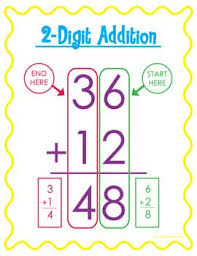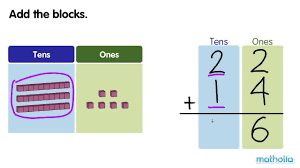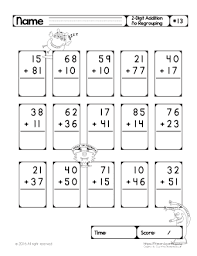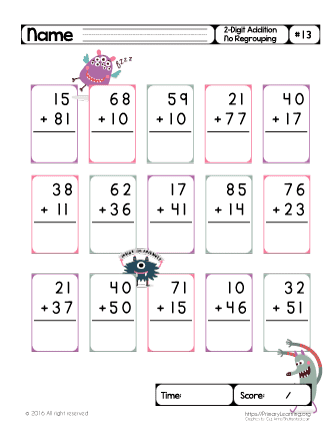HELLO CHILDREN, WELCOME BACK TO CLASS

SUBTOPIC: ADDITION OF 2-DIGIT NUMBERS WITHOUT REGROUPING

How to add two-digit numbers (for example 42 + 53).

Place one number above the other number so that the tens’ place digits and ones’ place digits are lined up. Draw a line under the bottom number.

4    2          =   40   + 2

+   5    3               50   + 3

————-            ————-

————–           ————-

Add the two unit’ place digits. (2 + 3 = 5). Place the five below the line in the ones’ place column.

4     2

+   5    3

—————

5

Add the numbers in the tens’ place column (4 + 5 = 9) and place the answer below the line and to the left of the ones’ place sum.

4    2                      = 40 + 2

+   5    3                         50 + 3

————-                    —————-

9    5                         90 + 5ACTIVITY

1. i) 26 + 73
2. ii) 30 + 35

iii)  23 + 41

1. iv) 43 + 20
2. v) 67 + 12
3. vi) 44  25Your Opinion Matters! Quickly tell us how to improve your Learning Experience HERE